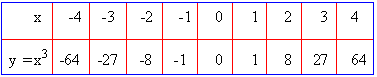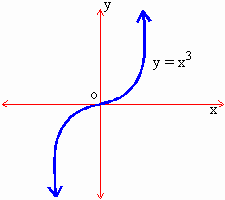Home MonkeyNotes Printable Notes Digital Library Study Guides Study Smart Parents Tips College Planning Test Prep Fun Zone Help / FAQ How to Cite New Title Request

5.7 Extreme Value Theorem

In a certain interval, it is possible to determine the maximum and minimum values of the function when we know the critical points of the function. The extreme value theorem guarantees both the maximum and minimum value of a function under certain conditions.

It is stated as:

If a function f (x) is continuous on a closed interval [a, b], then f (x) has both maximum and minimum value on [ a, b]

Procedure

I) First establish the continuity of f (x) on [a, b]

II) Determine all critical points of f (x) in [a, b]

III) Evaluate the function f (x) at these critical points and at the extremities of [a, b]

IV) The largest value is the maximum value and the smallest value is the minimum value of f (x) on [a, b]

Example 6

I) If f ' (x) > 0 for all x in the interval I, then 'f ' is an increasing in I and

II) If f ' (x) < 0 for all x in I then 'f ' is decreasing function in I. Is the converse of these two rules true?

Solution : Consider the curve y = x3 as shown in the figure.Your browser does not support the IFRAME tag.From the graph the curve is increasing at x = 0. But f ' (0) =0 i.e. f ' (0) is not positive Hence the converse of (1) is not true. In a similar way by considering y = x3 at x = 0, we can show that the curve is decreasing at x = 0 but f ' (0) = 0. Hence the curve decreasing at x = 0 but f ' (0) is not negative i.e. converse of (II) is also true.

Thus f ' ( c ) > 0 is only a sufficient condition for f (x) to be increasing at x = c and f ' (c) < 0 is only a sufficient condition for f (x) to be decreasing at x = c but it is not a necessary condition.

Index

5.1 Tangent And Normal Lines
5.2 Angle Between Two Curves
5.3 Interpretation Of The Sign Of The Derivative
5.4 Locality Increasing Or Decreasing Functions 5.5 Critical Points
5.6 Turning Points
5.7 Extreme Value Theorem
5.8 The Mean-value Theorem
5.9 First Derivative Test For Local Extrema
5.10 Second Derivative Test For Local Extrema
5.11 Stationary Points
5.12 Concavity And Points Of Inflection
5.13 Rate Measure (distance, Velocity And Acceleration)
5.14 Related Rates
5.15 Differentials : Errors And Approximation